# Table Api & SQL连续查询Join

Join是批处理数据处理中常见且易于理解的操作，用于连接两个关系的行。 但是，动态表上的连接语义不太明显甚至令人困惑。

## 常规JOIN

``````SELECT * FROM Orders
INNER JOIN Product
ON Orders.productId = Product.id
``````

### 时间窗口JOIN

``````SELECT *
FROM
Orders o,
Shipments s
WHERE o.id = s.orderId AND
o.ordertime BETWEEN s.shiptime - INTERVAL '4' HOUR AND s.shiptime
``````

## 时态表函数JOIN

``````SELECT * FROM Orders;

rowtime amount currency
======= ====== =========
10:15        2 Euro
10:30        1 US Dollar
10:32       50 Yen
10:52        3 Euro
11:04        5 US Dollar
``````

RatesHistory代表一个不断变化的仅追加货币汇率表，相对于日元（汇率为1）。 例如，欧元兑日元从09:00到10:45的汇率为114.从10:45到11:15，汇率为116。

``````SELECT * FROM RatesHistory;

rowtime currency   rate
======= ======== ======
09:00   US Dollar   102
09:00   Euro        114
09:00   Yen           1
10:45   Euro        116
11:15   Euro        119
11:49   Pounds      108
``````

``````rowtime amount currency
======= ====== =========
10:15        2 Euro
``````

``````SELECT
SUM(o.amount * r.rate) AS amount
FROM Orders AS o,
RatesHistory AS r
WHERE r.currency = o.currency
AND r.rowtime = (
SELECT MAX(rowtime)
FROM RatesHistory AS r2
WHERE r2.currency = o.currency
AND r2.rowtime <= o.rowtime);
``````

``````SELECT
o.amount * r.rate AS amount
FROM
Orders AS o,
LATERAL TABLE (Rates(o.rowtime)) AS r
WHERE r.currency = o.currency
``````

### 用法

``````SQL：
SELECT
SUM(o_amount * r_rate) AS amount
FROM
Orders,
LATERAL TABLE (Rates(o_proctime))
WHERE
r_currency = o_currency

JAVA:
Table result = orders
.join(new Table(tEnv, "rates(o_proctime)"), "o_currency = r_currency")
.select("(o_amount * r_rate).sum as amount");

SCALA:
val result = orders
.join(rates('o_proctime), 'r_currency === 'o_currency) .select(('o_amount * 'r_rate).sum as 'amount)

``````

## 与时态表JOIN

LatestRates是使用最新汇率实现的维度表。在时间10：15、10：30、10：52，LatestRates的内容如下：

``````10:15> SELECT * FROM LatestRates;

currency   rate
======== ======
US Dollar   102
Euro        114
Yen           1

10:30> SELECT * FROM LatestRates;

currency   rate
======== ======
US Dollar   102
Euro        114
Yen           1

10:52> SELECT * FROM LatestRates;

currency   rate
======== ======
US Dollar   102
Euro        116     <==== changed from 114 to 116
Yen           1
``````

``````SELECT * FROM Orders;

amount currency
====== =========
2 Euro             <== arrived at time 10:15
1 US Dollar        <== arrived at time 10:30
2 Euro             <== arrived at time 10:52
``````

``````amount currency     rate   amout*rate
====== ========= ======= ============
2 Euro          114          228    <== arrived at time 10:15
1 US Dollar     102          102    <== arrived at time 10:30
2 Euro          116          232    <== arrived at time 10:52
``````

``````SELECT
o.amout, o.currency, r.rate, o.amount * r.rate
FROM
Orders AS o
JOIN LatestRates FOR SYSTEM_TIME AS OF o.proctime AS r
ON r.currency = o.currency
``````

• 时态表函数join的SQL语法是联接UDTF，而时态表join使用SQL：2011中引入的标准时态表语法。
• 时态表函数join的实现实际上联接了两个流并使它们保持状态，而时态表join仅接收唯一的输入流并根据记录中的键查找外部数据库。
• 时态表函数hoin通常用于联接变更日志流，而时态表join通常用于联接外部表（即维表）。

### 用法

``````SELECT [column_list]
FROM table1 [AS <alias1>]
[LEFT] JOIN table2 FOR SYSTEM_TIME AS OF table1.proctime [AS <alias2>]
ON table1.column-name1 = table2.column-name1
``````

``````SELECT
SUM(o_amount * r_rate) AS amount
FROM
Orders
JOIN LatestRates FOR SYSTEM_TIME AS OF o_proctime
ON r_currency = o_currency
``````

2. 它仅在SQL中受支持，而在Table API中尚不支持。

##### 热门推荐
•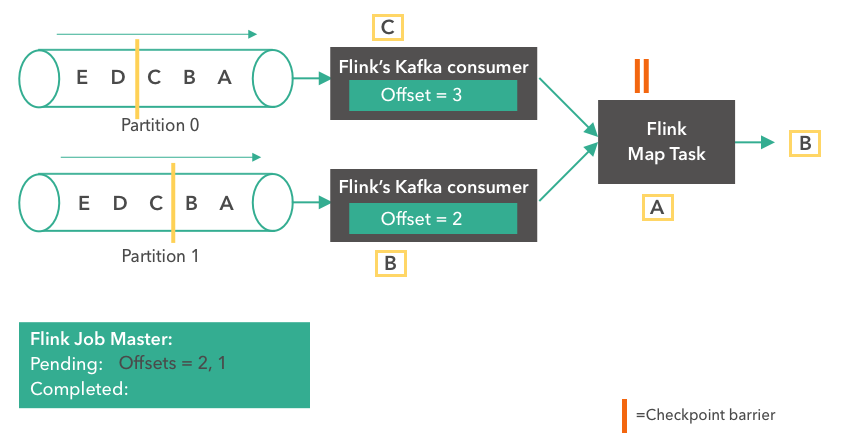浏览(1,615)
•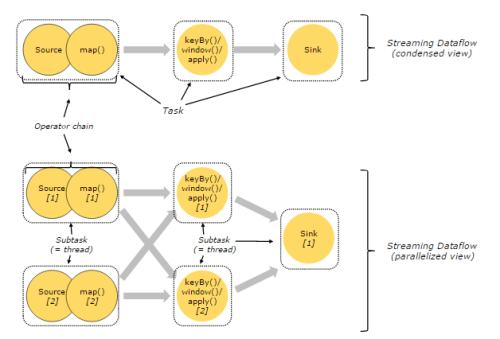浏览(1,358)
•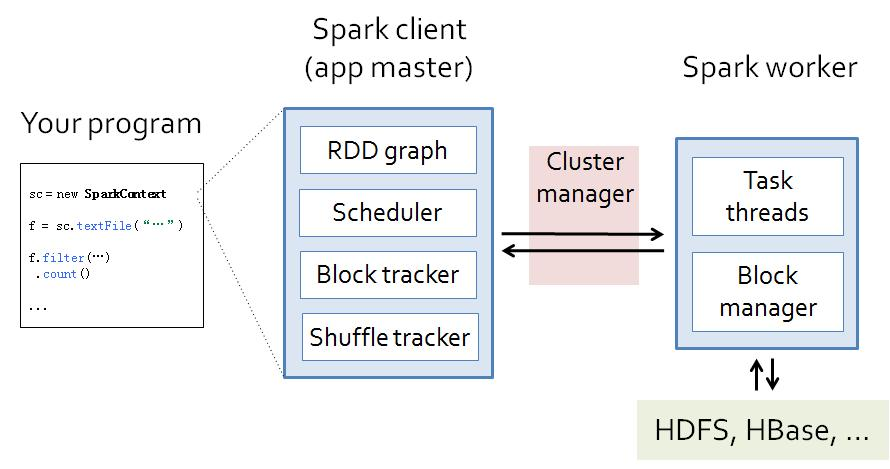浏览(1,348)
•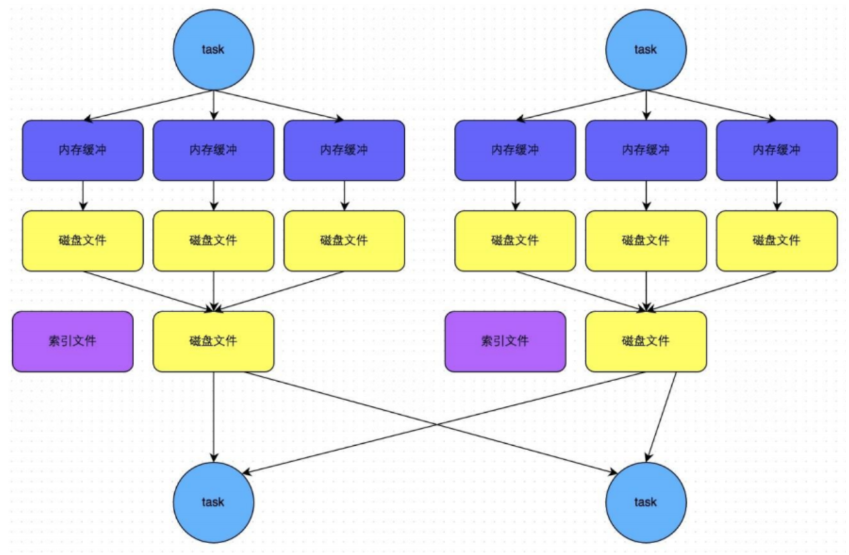浏览(1,291)
•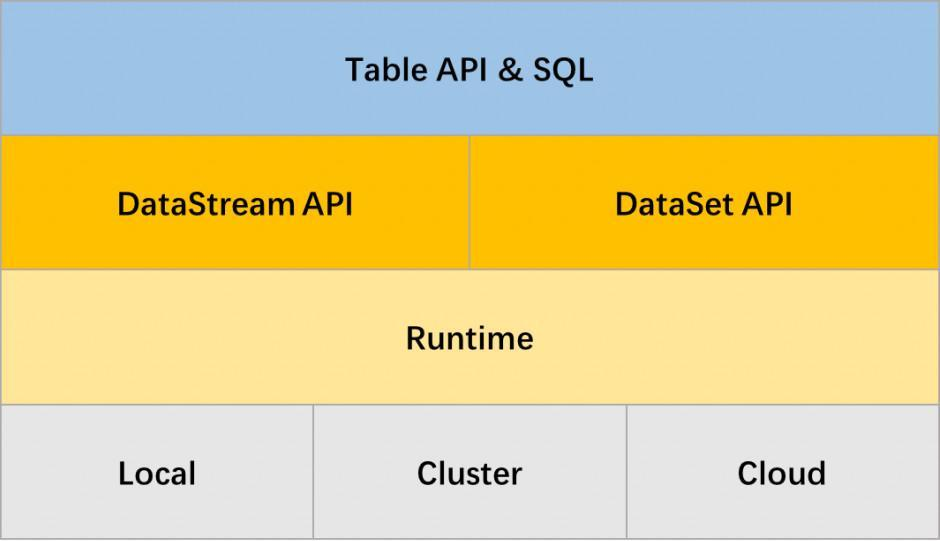浏览(1,227)
•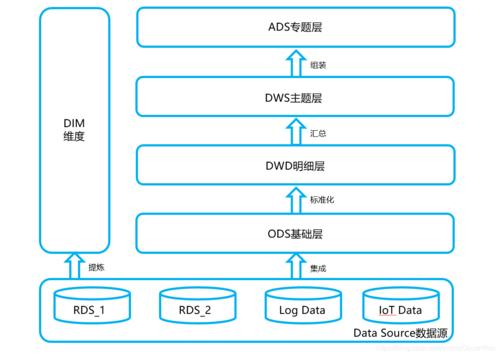浏览(1,176)
•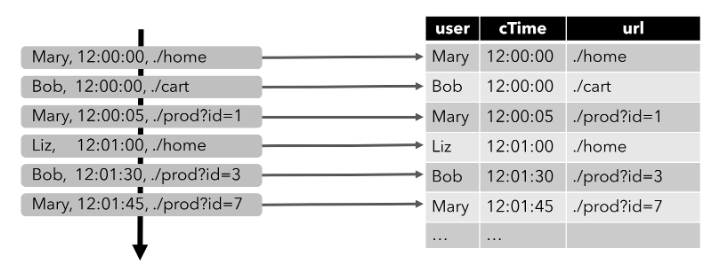浏览(1,167)
•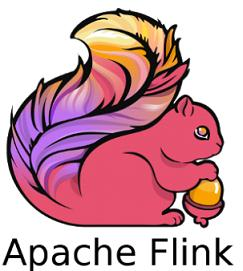浏览(1,140)
•浏览(1,094)
•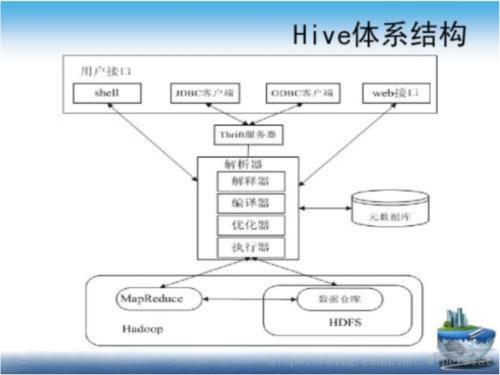浏览(1,081)